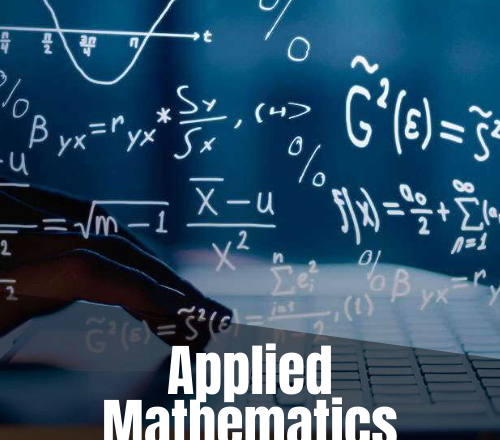# Applied Mathematics – 1 Tuition

4 out of 5
4
6 reviewsJoin The Best Applied Mathematics-1 Tuition Class. Academy Of Engineers Provides Best Tutorial Services For National And Foreign University. Digital Signal Processing Tuition Classes Are Available In Online As Well As Offline Mode. We Cover Syllabus Of All University. Applied Mathematics-1 Tuition Is The Common Subject Taught To The Students During First Year. Contact Us For The Best Online Applied Mathematics Tutor.

Objective: The objective of the paper is to facilitate the student with the basics of Applied Mathematics that are required for an engineering student

### Main Features

• Common For All B.Tech Students 1st year
• Basics of class 11th & 12th are required
• Classes are available in group as well as One-To-One
• Highly Experienced faculties from colleges and corporates
• Individual attention with free test series and doubt session
• 100% Passing Results
• Timing is flexible and adjustable
• Classes are available in online as well as offline mode

### Syllabus Of Applied Mathematics-1

Syllabus May Vary As Per University Curriculum

• Unit I : Successive differentiation: Leibnitz theorem for nth derivative (without proof). Infinite series: Convergence and divergence of infinite series, positive terms infinite series, necessary condition, comparison test (Limit test), D’Alembert ratio test, Integral Test, Cauchy’s root test, Raabe’s test and Logarithmic test(without proof). Alternating series, Leibnitz test, conditional and absolutely convergence. Taylor’s and Maclaurin’s expansion(without proof) of function ( ex , log(1+x), cos x , sin x) with remainder terms ,Taylor’s and Maclaurin’s series, Error and approximation.
• Unit II :

Asymptotes to Cartesian curves. Radius of curvature and curve tracing for Cartesian, parametric and polar curves. Integration: integration using reduction formula for. Application of integration: Area under the curve, length of the curve, volumes and surface area of solids of revolution about axis only. Gamma and Beta functions.

• UNIT- III : Matrices: Orthogonal matrix, Hermitian matrix, Skew-Hermitian matrix and Unitary matrix. Inverse of matrix by Gauss-Jordan Method (without proof). Rank of matrix by echelon and Normal (canonical) form. Linear dependence and linear independence of vectors. Consistency and inconsistency of linear system of homogeneous and non-homogeneous equations. Eigen values and Eigen vectors. Properties of Eigen values (without proof). Cayley-Hamilton theorem (without proof). Diagonalization of matrix. Quadratic form, reduction of quadratic form to canonical form.
• UNIT-IV : Ordinary differential equations: First order linear differential equations, Leibnitz and Bernaulli’s equation. Exact differential equations, Equations reducible to exact differential equations. Linear differential equation of higher order with constant coefficients, Homogeneous and non-homogeneous differential equations reducible to linear differential equations with constant coefficients. Method of variation of parameters. Bessel’s and Legendre’s equations (without series solutions), Bessel’s and Legendre’s functions and their properties.

Text:

[T1] B. S. Grewal,”Higher Engineering Mathematics” Khanna Publications.

[T2]. R. K. Jain and S.R.K. Iyengar,”Advanced Engineering Mathematics “Narosa Publications.

References:

[R1] E. kresyzig,” Advance Engineering Mathematics”, Wiley publications

[R2] G.Hadley, “ Linear Algebra” Narosa Publication

[R3] N.M. Kapoor, “ A Text Book of Differential Equations”, Pitambar publication.

[R4] Wylie R, “ Advance Engineering mathematics” , McGraw-Hill

[R5] Schaum’s Outline on Linear Algebra, Tata McGraw-Hill [R6] Polking and Arnold, “ Ordinary Differential Equation using MatLab” Pearson.

### Applied Mathematics -1

1
Applied Mathematics Tuition
2
Applied Mathematics Tuition

### Register Now By Calling 91-9818003202

Students of B.Tech 1st Year are elibigilble to attend this class
Purpose is to clear doubts and equip engineering students with the right concepts so that they can score high in semester exam.
We have a team of highly qualified faculties to teach all subjects of B.Tech Subjects.

### Fresh Batch is going to start soon

— 28 February 2017

1. Applied Mathematics-1(Engineering Mathematics-1) Stay focused towards your goal to achieve high success during semester exam. Start taking serious each and every exam along with assignment and project work!
2. Solve questions from different text books. Try to solve questions from different text book, utilize your time during zero period
3. Set Your Goal(Do it now) Set your goal from right from beginning and bring in hobbits to do it now mindset. I promise you will never be back in your career

4
4 out of 5
6 Ratings

#### Detailed Rating

 Stars 5 3 Stars 4 0 Stars 3 3 Stars 2 0 Stars 1 0

#### {{ review.user }}

{{ review.time }}